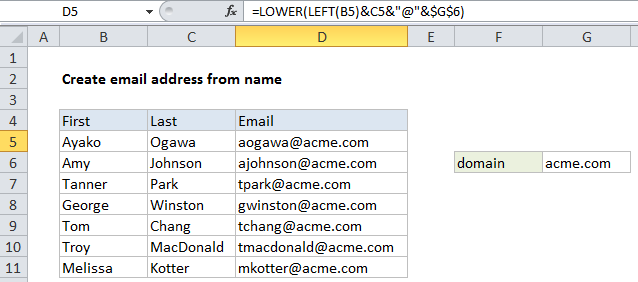## Excel Office

Excel How Tos, Tutorials, Tips & Tricks, Shortcuts

# How to create email address with name and domain in Excel

Atimes a user may want to build an email address from a first and last name. In that case, use a formula based on simple concatenation with help from the LEFT and LOWER functions. See illustration below:

## Formula

`=LOWER(LEFT(first)&last&"@"&domain)`## Explanation

In the example shown, the formula in D5 is:

`=LOWER(LEFT(B5)&C5&"@"&\$G\$6)`

### How this formula works

Working from the inside out, this formula first concatenates the last name in column C with the first letter of the first name from column B:

`LEFT(B5)&C5`

The LEFT function is normally configured with num_chars, but the argument is optional and left will extract 1 character from the left when it is absent. This results in the text string “atanaka”.

Worked Example:   How to get Excel workbook path only

Next, the domain name is added like this:

`"atanaka"&"@"&\$G\$6`

Note that the reference to the domain name is absolute (\$G\$6) so that the formula can be copied down the table without this reference changing.

Worked Example:   Remove first character in a cell in Excel

Finally, the lower function is used to lowercase the entire string:

`=LOWER("ATanaka@acme.com")`

which returns a final result of “atanaka@acme.com”

Worked Example:   How to Separate Text Strings in Excel